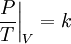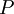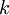# Gay-Lussac's law$\left. \frac{P}{T}\right\vert_V=k$
where$P$ is the pressure,$T$ is the temperature and$k$ is a constant. This holds true for an ideal gas.